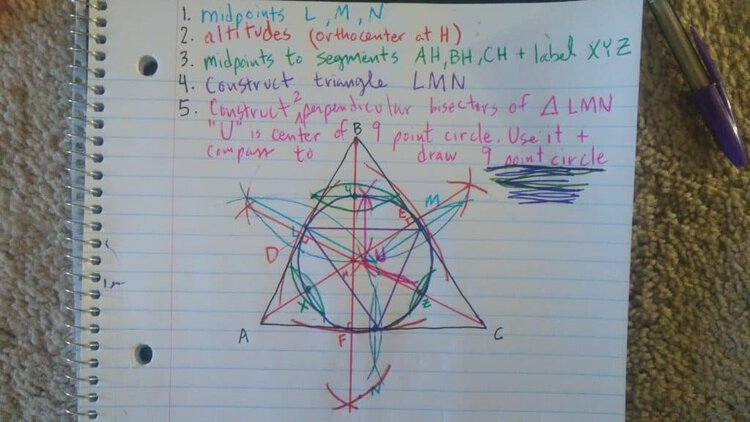# Constructing a 9 point circle

Break out your compass, ruler and colorful pens. Here’s the cliff notes version of constructing a 9 point circle by hand.

1.Draw a scalene triangle and construct midpoints. These are done by putting the sharp end of the compass on a vertex and extending the pencil slightly more than half the estimated distance along that segment of the triangle. Draw an arc and do it the same from the other end of the segment. Repeat for the other two sides.

2. Draw altitudes. Put sharp end of compass at a vertex opposite the line you’ll be bisecting. Draw and arc that crosses that segment twice. Then draw a perpendicular bisector of those two points by drawing arcs outside of the triangle, with the base of the compass at the points where the previous arc intersected the segment. Then draw a straight line from that point to the vertex of interest. Do two more altitudes from remaining vertices. Intersection point of all three altitudes is called orthocenter

3.Draw midpoints between orthocenter and vertices.

4.Construct an internal triangle with the vertices being the midpoints of each side of the original triangle.

5. Find the center of the 9 point circle by constructing two perpendicular bisectors of this new triangle. The intersection point of the bisecting lines i the center of the 9 point circle. Place sharp end of compass at center point, extend pencil to corner of the internal triangle and spin a circle.